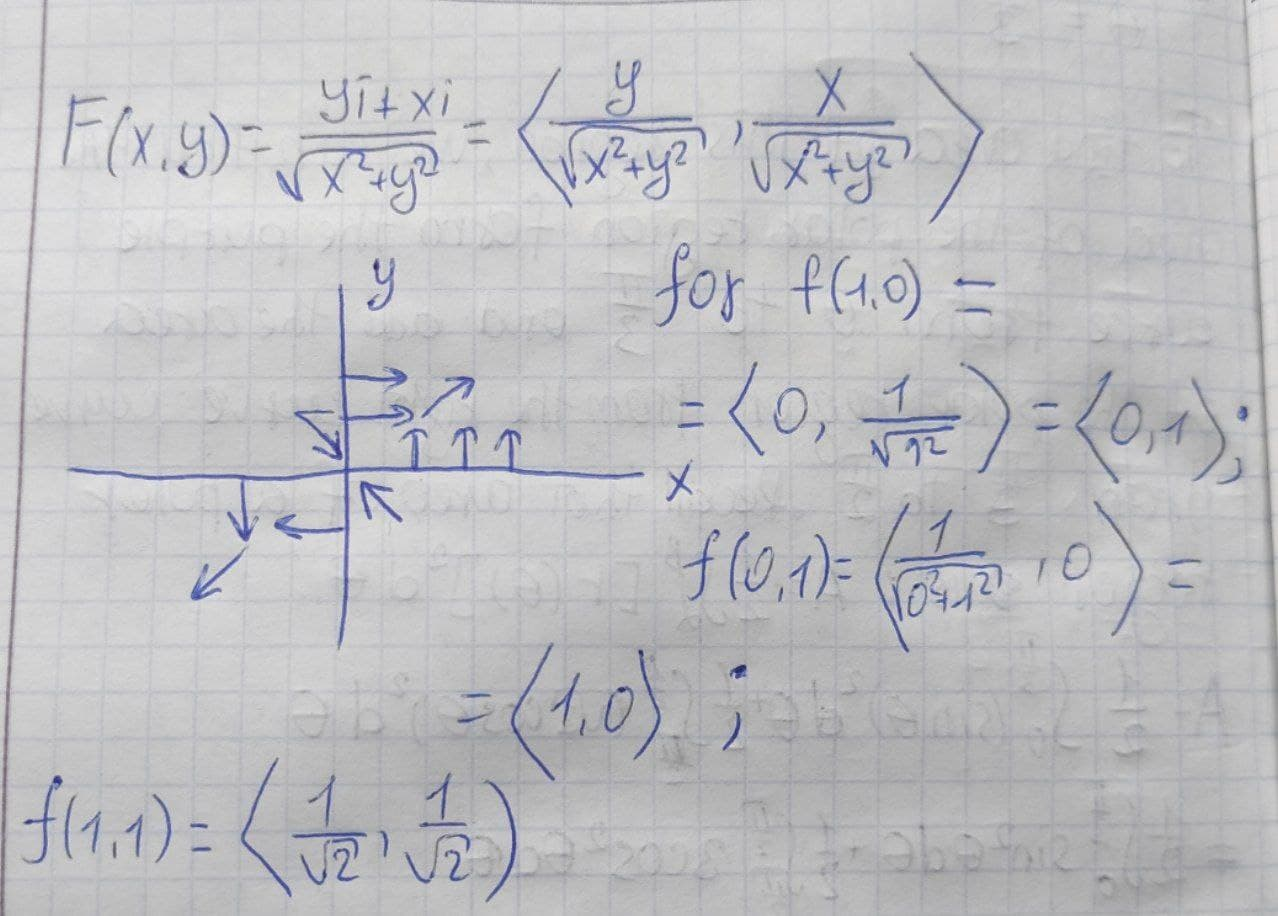# Sketch the vector field F by drawing a diagram. F(x,y)=frac{yi+xj}{(x^{2}+y^{2})^{frac{1}{2}}}Bevan Mcdonald 2021-09-15 Answered
Sketch the vector field F by drawing a diagram. $$\displaystyle{F}{\left({x},{y}\right)}={\frac{{{y}{i}+{x}{j}}}{{{\left({x}^{{{2}}}+{y}^{{{2}}}\right)}^{{{\frac{{{1}}}{{{2}}}}}}}}}$$

• Questions are typically answered in as fast as 30 minutes

### Plainmath recommends

• Get a detailed answer even on the hardest topics.
• Ask an expert for a step-by-step guidance to learn to do it yourself.Nichole Watt
Solution is on photo below